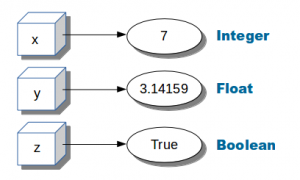# Tag: raw_input

## Python variablesVariables in Python (x,y,z). They can be used later in the program

Variables can hold numbers that you can use one or more times.

Numbers can be of one of these datatypes:

• integer (1,2,3,4)

• float (numbers behind the dot)

• boolean (True or False)

Related Course:
Complete Python Bootcamp: Go from zero to hero in Python 3## Numeric variables example

Example of numeric variables:

You can output them to the screen using the print() function.

Python supports arithmetic operations like addition (+), multiplication (*), division (/) and subtractions (-).

More mathematical operations

## User input

Python 3
Use the input() function to get text input, convert to a number using int() or float().

Python 2 (old version)
You can also ask the user for input using the raw_input function:

Download Python Exercises